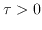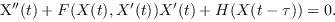## Cemil Tunç: Instability of solutions of vector Liénard equation with constant delay, p.197-204

### Abstract:

We consider the vector Liénard equation with the constant delay,We establish certain sufficient conditions, which guarantee the instability of the zero solution of this equation. An example is given to illustrate the theoretical analysis in this work.

Key Words: Vector Liénard equation, instability, delay.

2000 Mathematics Subject Classification: Primary: 34K12;
Secondary: 34K20.